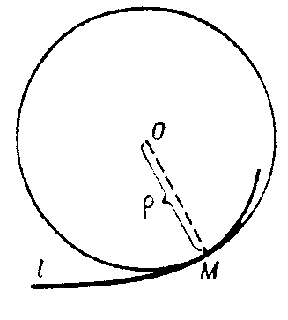# Osculating circle

at a given point $M$ of a curve $l$

The circle that has contact of order $n\geq2$ with $l$ at $M$ (see Osculation). If the curvature of $l$ at $M$ is zero, then the osculating circle degenerates into a straight line. The radius of the osculating circle is called the radius of curvature of $l$ at $M$, and its centre the centre of curvature (see Fig.). If $l$ is the plane curve given by an equation $y=f(x)$, then the radius of the osculating circle is given by

$$\rho=\left|\frac{(1+y'^2)^{3/2}}{y''}\right|.$$Figure: o070540a

If $l$ is the spatial curve given by equations

$$x=x(u),\quad y=y(u),\quad z=z(u),$$

then the radius of the osculating circle is given by

$$\rho=\frac{(x'^2+y'^2+z'^2)^{3/2}}{\sqrt{(y'z''-z'y'')^2+(z'x''-x'z'')^2+(x'y''-y'z'')^2}}$$

(where the primes denote differentiation with respect to $u$).